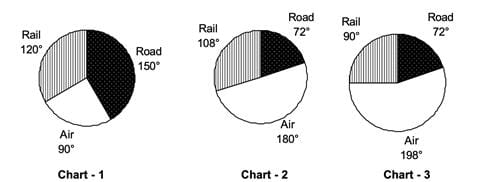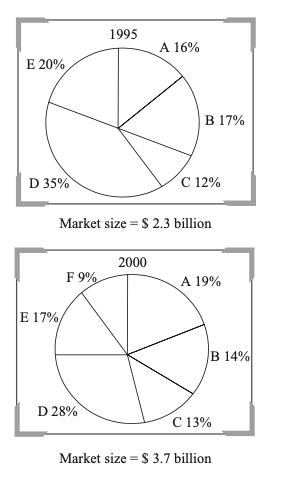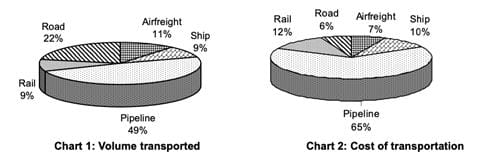Practice Test: Pie Chart - 2

# Practice Test: Pie Chart - 2

Test Description

## 10 Questions MCQ Test CSAT Preparation for UPSC CSE | Practice Test: Pie Chart - 2

Practice Test: Pie Chart - 2 for Banking Exams 2023 is part of CSAT Preparation for UPSC CSE preparation. The Practice Test: Pie Chart - 2 questions and answers have been prepared according to the Banking Exams exam syllabus.The Practice Test: Pie Chart - 2 MCQs are made for Banking Exams 2023 Exam. Find important definitions, questions, notes, meanings, examples, exercises, MCQs and online tests for Practice Test: Pie Chart - 2 below.
Solutions of Practice Test: Pie Chart - 2 questions in English are available as part of our CSAT Preparation for UPSC CSE for Banking Exams & Practice Test: Pie Chart - 2 solutions in Hindi for CSAT Preparation for UPSC CSE course. Download more important topics, notes, lectures and mock test series for Banking Exams Exam by signing up for free. Attempt Practice Test: Pie Chart - 2 | 10 questions in 10 minutes | Mock test for Banking Exams preparation | Free important questions MCQ to study CSAT Preparation for UPSC CSE for Banking Exams Exam | Download free PDF with solutions
 1 Crore+ students have signed up on EduRev. Have you?
Practice Test: Pie Chart - 2 - Question 1

### Answer the questions on the basis of the information given below. ABC Ltd. is a courier company that uses three modes of transport for its services – Air, Rail and Road. The following pie charts show the distribution of the total number of couriers (Chart-1), revenue generated (Chart-2) and total cost (Chart-3) for the different modes of transport for the year 2017. Assume that the only cost involved was that incurred on transportation.Q. In 2017, if the company made a profit of 20%, what was the profit percentage on the couriers sent by Air transport?

Detailed Solution for Practice Test: Pie Chart - 2 - Question 1

The ratio of total revenue to the total cost for
Air transport =10R/ 11C
Where R is the revenue generated by the company. C is the total cost of the company.
If the profit% of the company is 20%, then R/C = 1.2
So, total revenue as a fraction of cost for couriers
sent through Air transport = 10/11 X 1.2 = 1.0909
Hence, the profit% for couriers sent through
Air transport = 9.09%

Practice Test: Pie Chart - 2 - Question 2

### Answer the questions on the basis of the information given below. ABC Ltd. is a courier company that uses three modes of transport for its services – Air, Rail and Road. The following pie charts show the distribution of the total number of couriers (Chart-1), revenue generated (Chart-2) and total cost (Chart-3) for the different modes of transport for the year 2017. Assume that the only cost involved was that incurred on transportation.Q. Through which mode of transportation did the company generate maximum profit in 2017?

Detailed Solution for Practice Test: Pie Chart - 2 - Question 2

Let the total cost be 360C and the revenue generated be 360R for ABC Ltd. in the year 2017. Profit generated by:
Road transport = 72R – 72C.
Rail transport = 108R – 90C.
Air transport = 180R – 198C.
The profit generated is a function of R and C whose value are not known.
So the answer cannot be determined.

Practice Test: Pie Chart - 2 - Question 3

### Answer the questions on the basis of the information given below. ABC Ltd. is a courier company that uses three modes of transport for its services – Air, Rail and Road. The following pie charts show the distribution of the total number of couriers (Chart-1), revenue generated (Chart-2) and total cost (Chart-3) for the different modes of transport for the year 2017. Assume that the only cost involved was that incurred on transportation.Q. Which mode of transportation had the least cost of transport per courier in the year 2017 for the company?

Detailed Solution for Practice Test: Pie Chart - 2 - Question 3

Let the total number of couriers be 360N and the total cost be 360C. So the cost per courier for:
Road transport = 72C/150N = 0.48C/N
Rail transport = 90C /120N = 0.75C/N .
Air transport = 198C / 90N = 2.2C/N
It is clear from the above that the road transport has the least cost of transport per courier.

Practice Test: Pie Chart - 2 - Question 4

The pie charts given below represent the market share of different players in the electronics market in the year 1995 and 2000. A new player F has entered the market in the given period.Q. What is the percentage increase in the sales of A in the year 2000 over 1995?

Detailed Solution for Practice Test: Pie Chart - 2 - Question 4

There are two successive increases—First is the total increase of 60% approx. (\$ 3.7 bn in 2000 from \$ 2.3 billion in 1995) and second is the percentage share of A by 20% approx. Hence total percentage increase = 92%.

Practice Test: Pie Chart - 2 - Question 5

The pie charts given below represent the market share of different players in the electronics market in the year 1995 and 2000. A new player F has entered the market in the given period.Q. Find the CAGR with which the market has grown for the period 1995–2000?

Detailed Solution for Practice Test: Pie Chart - 2 - Question 5

Market size in 2000 = \$ 3.7 billion
Market size in 1995 = \$ 2.3 billion
Therefore, growth rate
= [(3.7/2.3)1/5 – 1] × 100 = 10 %
Hence, option (b) is the correct answer.
[3.7/2.3 = 1.6, now to find (1.6)1/5 , 1.13 = 1.331 and 1.12 = 1.21 and 1.3 × 1.2 = 1.6
Thus, 1.61/5 = 1.1]

Practice Test: Pie Chart - 2 - Question 6

The pie charts given below represent the market share of different players in the electronics market in the year 1995 and 2000. A new player F has entered the market in the given period.Q. If the new entrant F has grown at the expense of B, C, and E, what would be the change in the net value of sales of E in the year 2000, had F not entered the market?

Detailed Solution for Practice Test: Pie Chart - 2 - Question 6

Since we do not know what part of market share of B. C and E has been eaten up by F, so cannot be determined.

Practice Test: Pie Chart - 2 - Question 7

The pie charts given below represent the market share of different players in the electronics market in the year 1995 and 2000. A new player F has entered the market in the given period.Q. What is the increase in sales (in \$ million) of A, B and C put together, over the 5 years period?

Detailed Solution for Practice Test: Pie Chart - 2 - Question 7

Sales value for A + B + C in 2000 = (13 + 14 + 19)% of 3.7 billion = 46 % × 3.7 = 1.702 billion, Sales value for A + B + C in 1995 = (16 + 17 + 12) % of 2.3 billion = 1.035 billion.
Hence increase in sales value = 1703 – 1035 = \$ 668 million.Hence, option (c) is the correct answer.

Practice Test: Pie Chart - 2 - Question 8

Answer the questions based on the pie charts given below.

Chart 1 shows the distribution of 12 million tonnes of crude oil transported through different modes over a specific period of time. Chart 2 shows the distribution of the cost of transporting this crude oil. The total cost was Rs. 30 million.Q. The cost in rupees per tonne of oil moved by rail and road happens to be roughly

Detailed Solution for Practice Test: Pie Chart - 2 - Question 8

Cost in rupees of oil moved by rail and road is 18% of 30 million = 5.4 million.
Volume of oil transported by rail and road
= 31% of 12 million tonnes = 3.72 million tonnes.
Cost in rupees per tonnes =5.4/3.72 =  1.5 approximately.

Practice Test: Pie Chart - 2 - Question 9

Answer the questions based on the pie charts given below.

Chart 1 shows the distribution of 12 million tonnes of crude oil transported through different modes over a specific period of time. Chart 2 shows the distribution of the cost of transporting this crude oil. The total cost was Rs. 30 million.Q. From the charts given, it appears that the cheapest mode of transport is

Detailed Solution for Practice Test: Pie Chart - 2 - Question 9

From the chart, we can make out the least among road, rail, pipeline, ship by looking at the ratio of cost to volume.
Rail= 12/9
Pipeline = 65 /49
Ship= 10/ 9
Since ratio of cost to volume for road is the lowest, it is the cheapest

Practice Test: Pie Chart - 2 - Question 10

Answer the questions based on the pie charts given below.

Chart 1 shows the distribution of 12 million tonnes of crude oil transported through different modes over a specific period of time. Chart 2 shows the distribution of the cost of transporting this crude oil. The total cost was Rs. 30 million.Q. If the costs per tonne of transport by ship, air and road are represented by P, Q and R respectively, which of the following is true?

Detailed Solution for Practice Test: Pie Chart - 2 - Question 10

Like the previous answer again look at the ratio of
10/9, 7/11, 6/22
So 10/9> 7/11 > 6/22
Hence, P>Q>R

## CSAT Preparation for UPSC CSE

93 videos|78 docs|107 tests
 Use Code STAYHOME200 and get INR 200 additional OFF Use Coupon Code
Information about Practice Test: Pie Chart - 2 Page
In this test you can find the Exam questions for Practice Test: Pie Chart - 2 solved & explained in the simplest way possible. Besides giving Questions and answers for Practice Test: Pie Chart - 2, EduRev gives you an ample number of Online tests for practice

## CSAT Preparation for UPSC CSE

93 videos|78 docs|107 tests